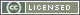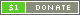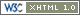# Logic

Instructor: Max Schaefer

This lecture aims to provide students with the logical background knowledge needed to understand and appreciate the other lectures.

None

# Syllabus

• Classical logic (propositional, first order) and its semantics
• Intuitionistic logic (propositional, first order), natural deduction, basic proof theory
• Equational logic, first order logic with equality, basic model theory

# Course Materials

1. First Lecture (July 2): slides, handout,homework, solutions
2. Second Lecture (July 3): slides, handout, homework, solutions
3. Third Lecture (July 9): slides, handout, homework, solutions
4. Exam (July 13): problems, solutions

Here are some supplementary exercises to be discussed after class on July 11.

# Tools

You can use NJEdit to construct derivations in natural deduction. Currently, NJEdit is only tested with Firefox, Opera, and Safari; it does not work with Internet Explorer, as demonstrated during the tutorial.